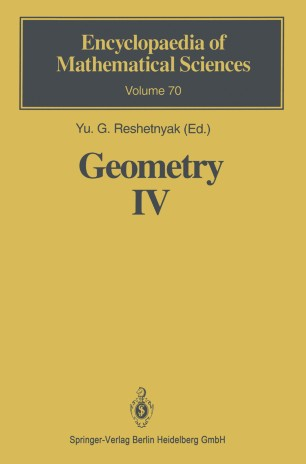# Geometry IV

## Non-regular Riemannian Geometry

• Yu. G. ReshetnyakBook

Part of the Encyclopaedia of Mathematical Sciences book series (EMS, volume 70)

1. Front Matter
Pages i-2
2. Yu. G. Reshetnyak
Pages 3-163
3. V. N. Berestovskij, I. G. Nikolaev
Pages 165-243
4. Back Matter
Pages 245-252

### Introduction

The book contains a survey of research on non-regular Riemannian geome­ try, carried out mainly by Soviet authors. The beginning of this direction oc­ curred in the works of A. D. Aleksandrov on the intrinsic geometry of convex surfaces. For an arbitrary surface F, as is known, all those concepts that can be defined and facts that can be established by measuring the lengths of curves on the surface relate to intrinsic geometry. In the case considered in differential is defined by specifying its first geometry the intrinsic geometry of a surface fundamental form. If the surface F is non-regular, then instead of this form it is convenient to use the metric PF' defined as follows. For arbitrary points X, Y E F, PF(X, Y) is the greatest lower bound of the lengths of curves on the surface F joining the points X and Y. Specification of the metric PF uniquely determines the lengths of curves on the surface, and hence its intrinsic geometry. According to what we have said, the main object of research then appears as a metric space such that any two points of it can be joined by a curve of finite length, and the distance between them is equal to the greatest lower bound of the lengths of such curves. Spaces satisfying this condition are called spaces with intrinsic metric. Next we introduce metric spaces with intrinsic metric satisfying in one form or another the condition that the curvature is bounded.

### Keywords

Approximation durch Polyeder Beschränkte Krümmung Bounded Curvature Integralkrümmung K-Konkavität Manifolds Mannigfaltigkeiten Metric Spaces Metrische Räume Plateausches Problem Riemannian geometry Riemannsche Geometrie Riemannsche Räume curvature manifold

### Editors and affiliations

• Yu. G. Reshetnyak
• 1
1. 1.Institute of Mathematics, Siberian Branch of the RussianAcademy of SciencesNovosibirskRussia

### Bibliographic information

• DOI https://doi.org/10.1007/978-3-662-02897-1
• Copyright Information Springer-Verlag Berlin Heidelberg 1993
• Publisher Name Springer, Berlin, Heidelberg
• eBook Packages
• Print ISBN 978-3-642-08125-5
• Online ISBN 978-3-662-02897-1
• Series Print ISSN 0938-0396
• Buy this book on publisher's site